# Digital Communications: A Discrete-Time Approachby Michael Rice# Symbol Timing Synchronization for Binary Baseband PAM

## Introduction

The previous Binary Baseband PAM Simulink Exercise assumed the phase of the bit clock was known. That is, you knew where the symbols began and ended. Unfortunately, this is not the case in practice and the bit (or symbol) timing must be extracted from the received signal samples. This is accomplished using a bit (or symbol) timing synchronizer.

In this exercise, you will design a bit timing synchronizer for binary baseband PAM based on the PLL. There are three sample rates in play in this design, so you must be careful in assigning sample times to the simulink blocks. The detector, with its accompanying bit timing synchronizer, will be used to process the modulated sampled in the file bbtrdata.mat.

## Textbook References

Baseband PAM: Section 5.2, discrete-time realizations: Section 5.2.2, partial response pulse shapes: Section A.2, general discussion of timing synchronization: Section 8.1, discrete-time techniques for symbol timing synchronization for binary PAM: Section 8.4.

## Specifications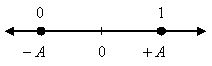normalized sample rate: 8 samples/bit average energy: 1 pulse shape: SRRC (50% excess bandwidth, span = 12 symbols) bit clock offset: unknown!! input file bbtrdata.mat packet format: TR = 1000 bits (you don't need to know what they are) SYNC = 1 0 1 1 0 1 0 0 1 0 1 1 0 1 0 0 DATA = 1288 bits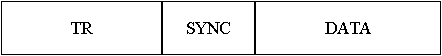## Preliminary Design

### Design the Detector

Design the detector, shown below, using blocks from the Simulink, DSP System, and Communications System Toolboxes.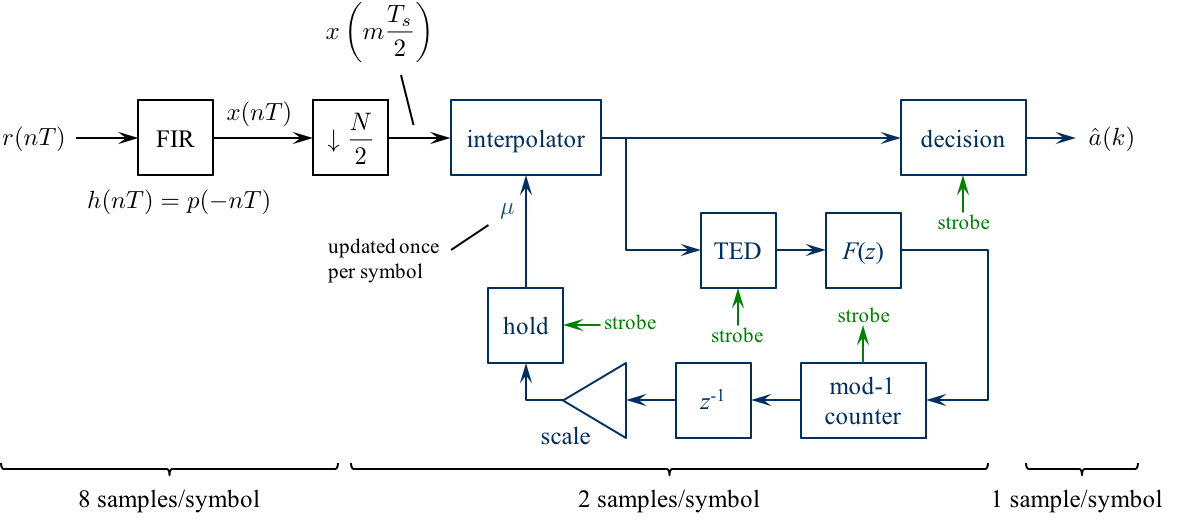Design the loop filter to create a second order loop to the following specifications: closed-loop equivalent noise bandwidth = 0.01 (normalized to the bit rate), damping factor = 0.7071.

### Design Notes

1. Because I plan on using the early-late detector for the timing error detector (TED) and the early-late TED operates at 2 samples/symbol, the matched filter output output is downsampled by 4. The matched filter and its input operate at 8 samples/symbol: the downsampled sequence is at 2 samples/symbol.

2. Use a Farrow interpolator. You can use either the piecewise-parabolic version (see Figure 8.4.17 on p. 437) or the cubic version (see Figure 8.4.18 on p. 438).

3. The strobe is used as an enable signal for the TED, decision, and mu update. The strobe signal is derived from the decrementing modulo-1 counter. Use the underflow condition in the decrementing mod-1 counter as the strobe.

4. You need to create an "enabled" version of the early-late TED. When the strobe is high, the TED output is given by equation (8.34) on page 420. When the strobe is low, the TED output is zero.

5. For interpolation control, use the decrementing modulo-1 counter described in Section 8.4.3 (pp. 441 - 444).

6. The "enabled hold" block outputs the previous value when the strobe is low, or passes the input to the output when the strobe is high. This block is needed because the fractional interval is updated by the mod-1 counter only once per symbol, but the interpolator operates at 2 samples/symbol. The hold operation ensures that the interpolator is using the proper value for the fractional interval.

Simulink provides a skeletal enabled subsystem:

Simulink -> Ports & Subsystems -> Enabled Sybsystem

The default enabled subsystem is shown below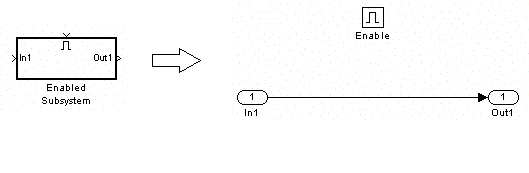The subsystem consists of a simple wire with the enable icon above it. This means the wire is only active when the enable signal exceeds a predefined threshold (double click on the enable block to set the threshold). When the enable signal is below the predefined threshold, nothing happens and the output holds its value.

7. The decision subsystem is also enabled. This is because decisions should be made on every other interpolator output on average. The enable signal indicates which interpolator outputs represent the desired signal space projections. An example of the enabled decision subsystem for binary PAM is shown below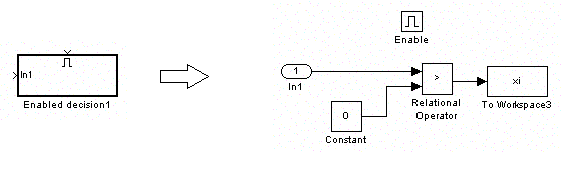## Exercise

1. Incorporate the symbol timing synchronization PLL into a binary PAM detector.

2. For the detector input, use the From File block and set the Filename to bbtrdata.mat and the sample time to 1.

3. Set the simulation parameters as follows:
 Simulation Time
 Start Time: 0.0 Stop Time: (2304+14)*8
Solver Options
 Type: Fixed-step Solver: discrete (no continuous states) Fixed step size: auto
Tasking and sample time options
 Periodic sample time constraint: Unconstrained Treat each discrete state as a separate task: Deselect

4. Run the simulation.

5. The detector produces many decisions. (The exact number depends on the number of times the strobe is high. This, in turn, depends on the dynamics of your symbol timing PLL.)

6. To find the data, look for the 16-bit SYNC pattern in the detector output. Once you find it, the following 1288 bit decisions correspond to 184 7-bit ASCII characters. Determine the message using either your Matlab script or an ASCII Table.

7. Plot the timing error (loop filter output) and use this plot to estimate how long (measured in bits) it took for your timing PLL to lock.

8. Plot the fractional interpolation interval (mu). What conclusions can you make based on this plot?
Brigham Young University - Provo | Fulton College of Engineering | The Church of Jesus Christ of Latter-day Saints
Department of Electrical and Computer Engineering, BYU, Provo, UT 84602 - (801)422-4012 - Copyright 2009. All Rights Reserved